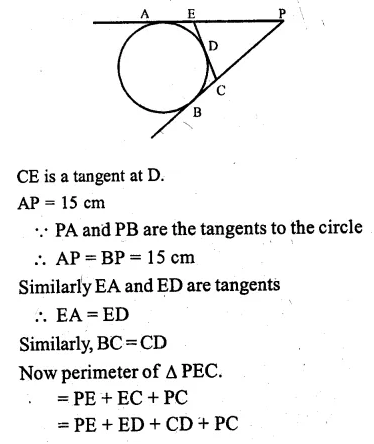Guru

# Question 8. (a) In the figure (i) given below, O is the centre of the circle and AB is a tangent at B. If AB = 15 cm and AC = 7.5 cm, find the radius of the circle. (b) In the figure (ii) given below, from an external point P, tangents PA and PB are drawn to a circle. CE is a tangent to the circle at D. If AP = 15 cm, find the perimeter of the triangle PEC.

• 0

This is circle based question from Chapter name- circles
Topic – Angle properties of circles
Chapter number- 15

It is given that , O is the centre of the circle and AB is a tangent at B. If AB = 15 cm and AC = 7.5 cm,

Then we have to find the radius of the circle. (b) In the figure given , from an external point P, tangents PA and PB are drawn to a circle.

It is also given that CE is a tangent to the circle at D. If AP = 15 cm,  now we have to find the perimeter of the triangle PEC.

ICSE Avichal publication
Understanding ICSE Mathematics
Question no 8

Share